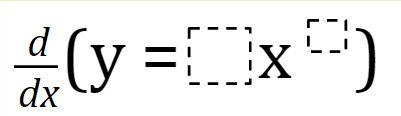Home > Calculus > Derivatives Power Rule 2

# Derivatives Power Rule 2

Directions: Using the digits 1 to 9 at most one time each, fill in the boxes to create a function such that at x = 2, the derivative (at that point) is closest to the value of 449.### Hint

Which blank has the LARGEST effect on the output?

How does the POWER RULE help you to get a possible solution?